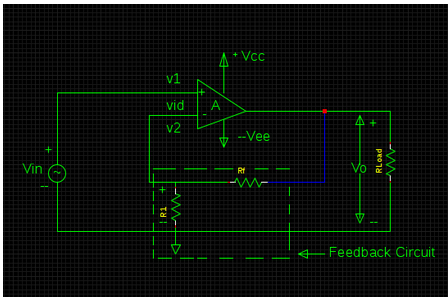# Numerical on Non-Inverting Amplifier.

## The below drawn schematic of Op-amp 741 is configured in non-inverting mode with R1= 2 K Ω ,Rf = 20 K Ω , fo = 10 Hz & A = 400,000. Determine the following parameters :1) Af 2)f Fa. 10.99 & 90.9 kHz
b. 10.99 & 36.36 kHz
c. 10.99 & 20 kHz
d. 10.99 & 10 kHz

Correct Answer : b. 10.99 & 36.36 kHz

Explanation :
For non-inverting mode of an amplifier (voltage series feedback amplifier)

1) Af = AB / 1+ AB
But, B = R1 / ( R1 + Rf) = 2 k / (2 k + 20 k)
= 1 / 11
1+ AB = 1 + 400000 x (1 / 11)
= 36364.6
Af = 400000 / 3636.4 = 10.99

2) fF = ( 1 + AB ) fo = ( 36364.6 ) x 10Hz = 363646 = 36.36 KHz